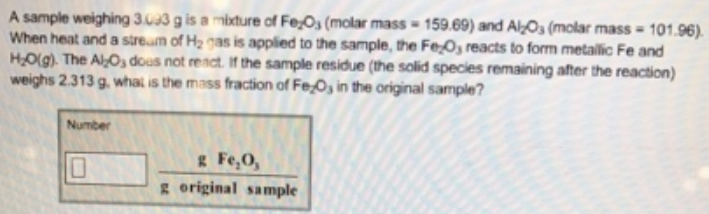# Problem: A sample weighing 3.093 g is a mixture of Fe 2O3 (molar mass = 159.69) and Al 2O3 (molar mass = 101.96). When heat and a stream of H2 gas is applied to the sample, the Fe2O3 reacts to form metallic Fe and H2O(g). The Al2O3 does not react. If the sample residue (the solid species remaining after the reaction) weighs 2.313 g, what is the mass fraction of Fe2O3 in the original sample?

###### FREE Expert Solution
93% (256 ratings)###### Problem Details

A sample weighing 3.093 g is a mixture of Fe 2O3 (molar mass = 159.69) and Al 2O3 (molar mass = 101.96). When heat and a stream of H2 gas is applied to the sample, the Fe2O3 reacts to form metallic Fe and H2O(g). The Al2O3 does not react. If the sample residue (the solid species remaining after the reaction) weighs 2.313 g, what is the mass fraction of Fe2O3 in the original sample?